Support the Monkey! Tell All your Friends and TeachersHome MonkeyNotes Printable Notes Digital Library Study Guides Study Smart Parents Tips College Planning Test Prep Fun Zone Help / FAQ How to Cite Request a New Title

4.3 Properties Of Arithmetic Mean

1. The sum of the deviations, of all the values of x, from their arithmetic mean, is zero.

Justification :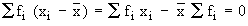Sinceis a constant,2. The product of the arithmetic mean and the number of items gives the total of all items.

Justification :or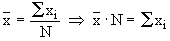3. If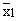and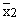are the arithmetic mean of two samples of sizes n1 and n2 respectively then, the arithmetic meanof the distribution combining the two can be calculated asThis formula can be extended for still more groups or samples.Justification := total of the observations of the first sample

Similarly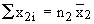= total of the observations of the first sample

The combined mean of the two samples

=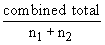=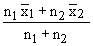Your browser does not support the IFRAME tag.
Example The average marks of three batches of students having 70, 50 and 30 students respectively are 50, 55 and 45. Find the average marks of all the 150 students, taken together.

Solution :

Let x be the average marks of all 150 students taken together.

Batch - I   Batch - II   Batch - III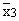A. marks :           = 50           = 55            = 45

No. of students n1 = 70    n2 = 50       n3    = 30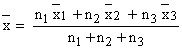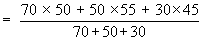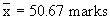Index

4.1 Introduction
4.2 Arithmetic Mean
4.3 Properties of Arithmetic Mean
4.4 Median
4.5 Mode
4.6 Empirical relation between mean, median & mode

Chapter 5All Contents Copyright © All rights reserved.
Further Distribution Is Strictly Prohibited.

 Search: All Products Books Popular Music Classical Music Video DVD Toys & Games Electronics Software Tools & Hardware Outdoor Living Kitchen & Housewares Camera & Photo Cell Phones Keywords: